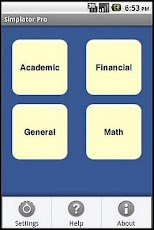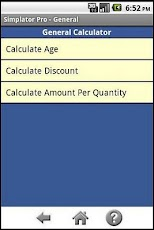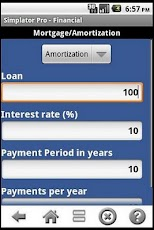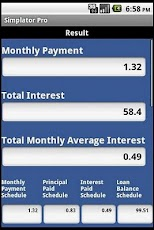# Simplator ProAdd to My Apps
Price \$0.99 Productivity 1.1 TOT The Orion Thetas 97 views 0 votes 0

Simplator Pro – Simple utility calculator that computes commonly used calculations e.g. salary, mortgage, amortization, discount, age, percentage calculation, number conversions (binary, decimal, hexadecimal), CGPA, GPA/SGPA and amount per quantity.No matter what you do in your daily life, Simplator Pro has functions that you will find handy!* Easy to use Interface.* 60+ calculations.* No advertisements.-> Calculations distributed among 4 CategoriesAcademic: Calculate CGPA, GPA, SGPA and Marks percentageFinancial: Calculate Salary, Mortgage & AmortizationGeneral: Calculate Age, Discount, Amount per QuantityMath: Calculate Percentage, Number Conversion, Measure Conversion-> Number Conversion supports conversions among Binary, Hexadecimal and Decimal numbers.-> Measure Conversion supports conversions with builtin Length and Weight calculatorsLength Calculator1. centimeter (cm) 2. feet (ft) 3. inch (in) 4. kilometer (km) 5. meter (m) 6. millimeter (mm) 7. mile (mi) 8. yard (yd) Weight Calculator1. ounce (oz) 2. pound (lb) 3. gram (gm) 4. kilogram (kg) 5. ton (ton)

## App Screenshots## Other Productivity Apps#### Dayhaps

(4)#### Androidden Para Kazan...

(8)#### Time It!

(4)#### Jennifer Lawrence Cal...

(3)#### Multiling O keyboard ...

(337)(6)